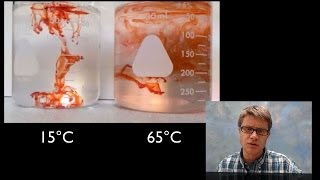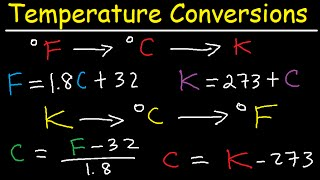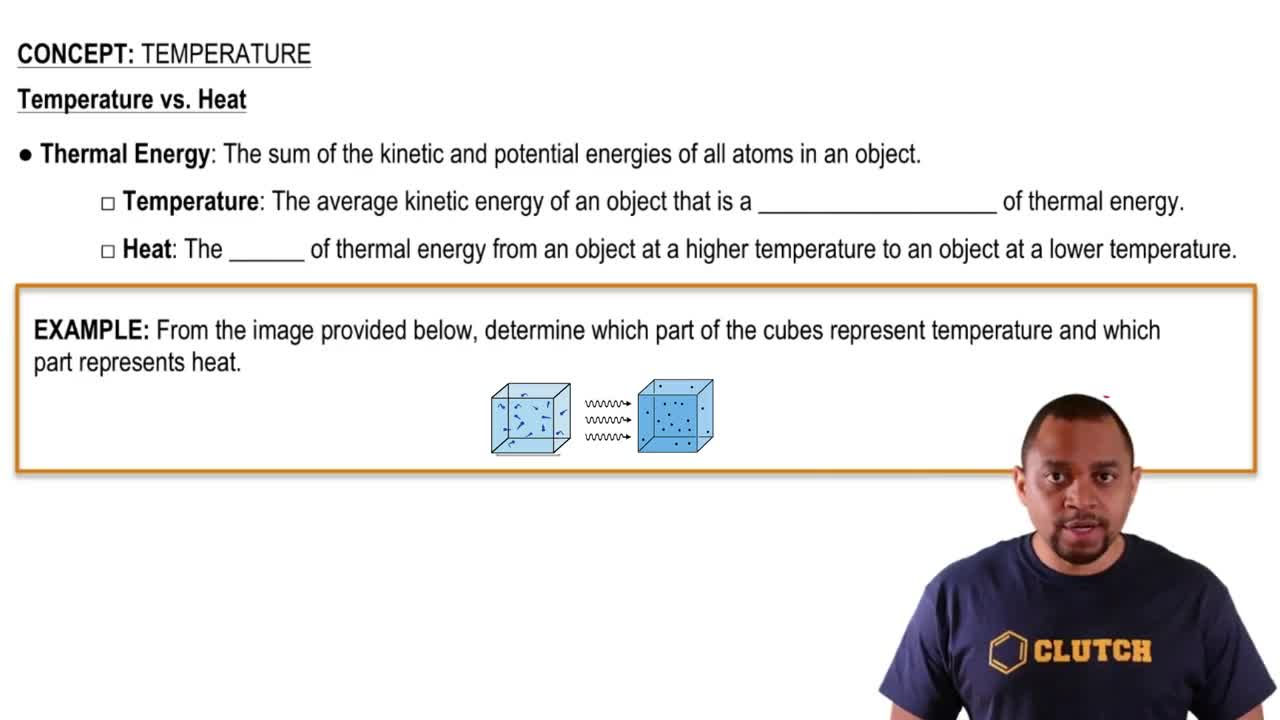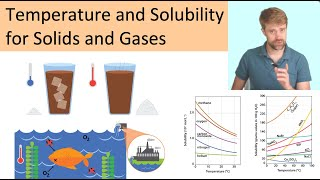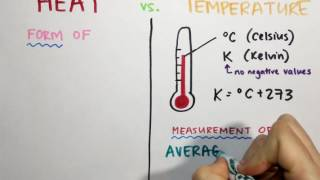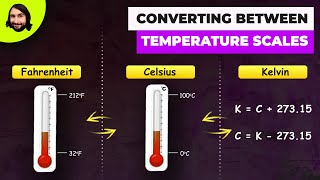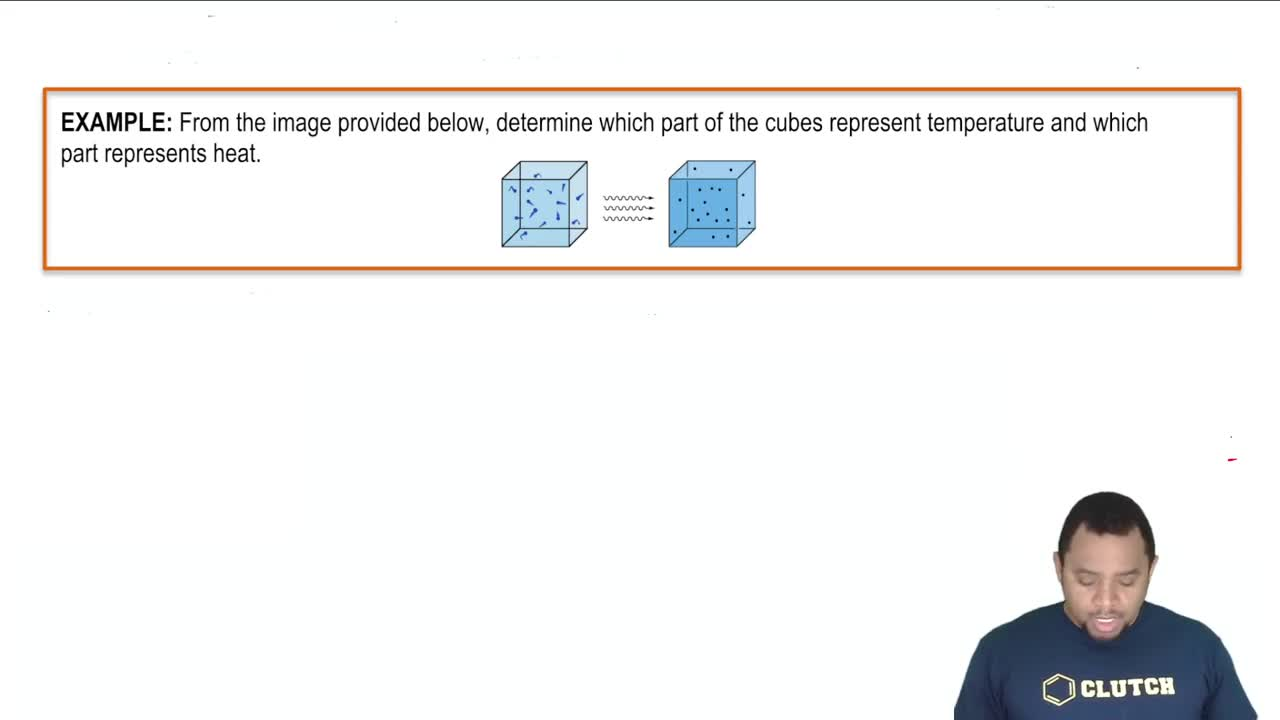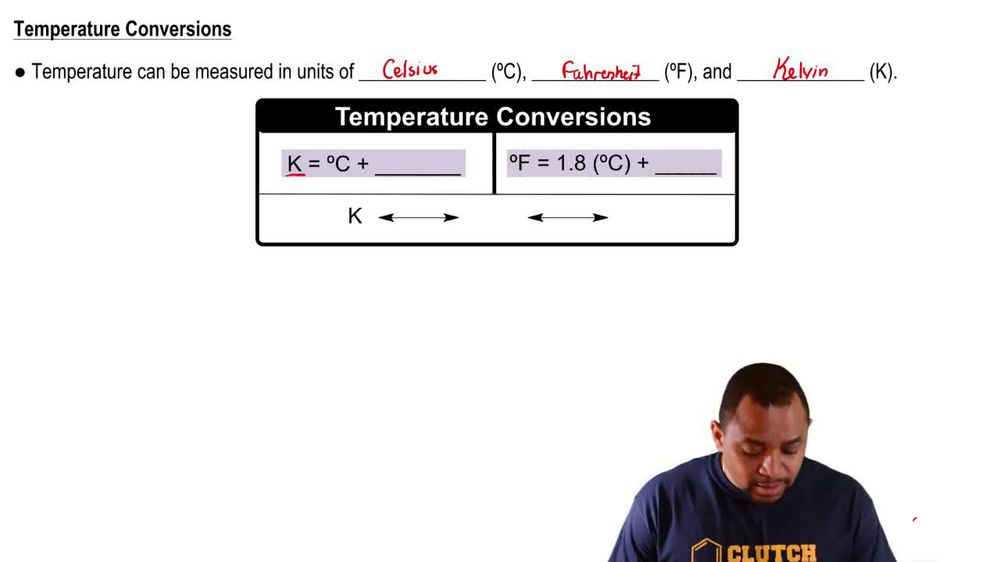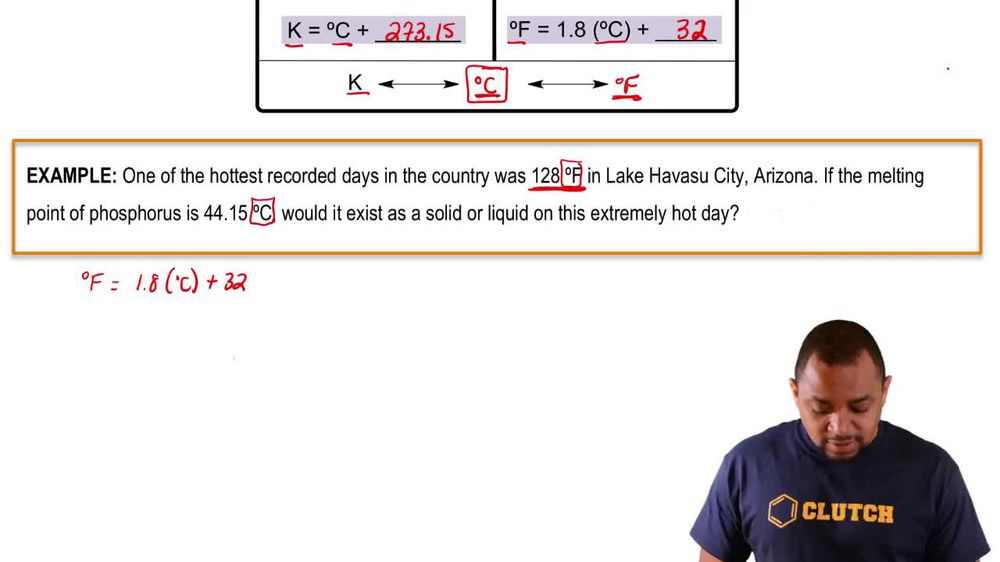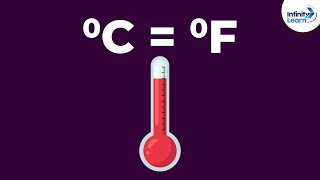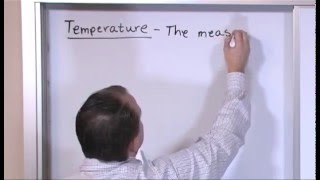Start typing, then use the up and down arrows to select an option from the list.
1. 1. Intro to General Chemistry2. Temperature
Problem

# At what temperatures are the readings on the Fahrenheit and Celsius thermometers the same?

Relevant Solution1m
Play a video:
Hey everyone, we're being asked to determine the temperature reading at which the Fahrenheit and Celsius thermometers are exactly the same for this question. We're going to need to recall the following equation. We know that degrees Fahrenheit is equal to 9/5°C plus 32. Now we want to change our degrees Fahrenheit and degrees Celsius into one variable and solve for that variable. And that's because we're looking for the temperature in which they are exactly the same. So doing so we end up with a variable T for temperature is equal to 1.8 and that's because 9/5 in decimal form is 1. T plus 32. Now, solving for T, we're going to Subtract 1.80 on both sides and don't forget that we have this invisible one right here behind our tea And we end up with negative 0.8 e equals 32. Now, all we need to do is divide our negative 0.8 onto both sides and we end up with a t of negative degrees. So this is going to be the temperature in which both Fahrenheit and Celsius thermometers are going to be the same. So I hope that made sense. And let us know if you have any questions